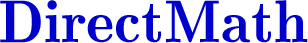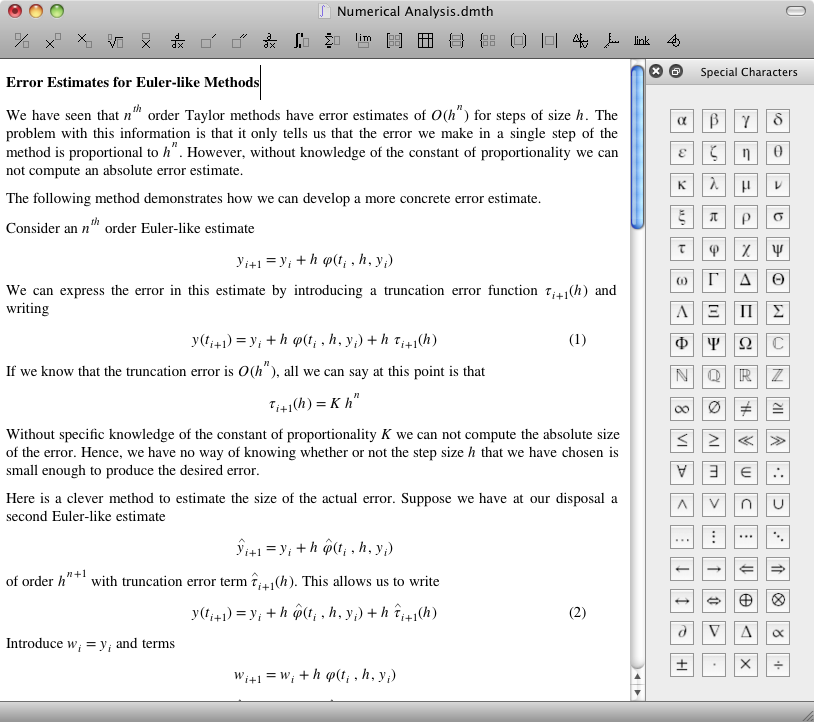#### Three applications in one

DirectMath is a combination of an easy to use mathematical editor, a computation system, and a collection of powerful technical drawing tools.

#### A mathematical editor

The DirectMath editor is a simple, streamlined editor optimized for creating and publishing mathematical content.Key features of the editor include

• Tool buttons that make it easy to create mathematical constructs such as integrals and matrices
• Symbol pallete that provides easy access to common mathematical symbols
• An editor designed from the ground up to work with the STIX fonts, a high quality set of fonts optimized for mathematical text
• A slide show feature that makes it easy to generate and display a slide show from a document
• Easy export to HTML and LaTeX

DirectMath produces high quality documents in PDF form and HTML form.

#### A powerful and easy to use computation system

DirectMath is a front end for the Mathematica and Maxima computer algebra systems. DirectMath uses a powerful built-in pattern matching system to anticipate the computations you want to do, making it possible for you to perform a wide range of operations without having to learn a specialized programming language. In particular, DirectMath makes it possible to do mathematical calculations in a semi-automated way, with user actions controling the computer algebra system.

#### Built-in drawing tools

DirectMath includes a set of drawing tools that allow you create sophisticated "live" drawings to illustrate mathematical concepts. The drawing tools couple simple and easy to use standard drawing tools with a powerful system of constraints. This allows you to create drawings that express mathematical ideas in a precise way. Drawings with constraints update automatically as you move parts of the drawing around.

#### About the author

DirectMath is developed by Joe Gregg, who is an associate professor of Math and Computer Science at Lawrence University in Appleton, Wisconsin. Joe Gregg has been working with Mathematica since 1989, and started developing software in 1993 in an effort to make computer algebra systems more accessible for students and teachers alike. Prof. Gregg uses DirectMath actively to teach courses in numerical analysis, differential equations, and computer science.